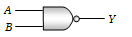The output of OR gate is 1

(a) If both inputs are zero

(b) If either or both inputs are 1

(c) Only if both input are 1

(d) If either input is zero

Concept Questions :-

Logic gates
High Yielding Test Series + Question Bank - NEET 2020

Difficulty Level:

Which gates is represented by this figure(a) NAND gate
(b) AND gate
(c) NOT gate
(d) OR gate

Concept Questions :-

Logic gates
High Yielding Test Series + Question Bank - NEET 2020

Difficulty Level:

Which of the following logic gate is an universal gate?

1. OR

2. NOT

3. AND

4. NOR

Concept Questions :-

Logic gates
High Yielding Test Series + Question Bank - NEET 2020

Difficulty Level:

In a crystal, the atoms are located at the position of

(a) Maximum potential energy

(b) Minimum potential energy

(c) Zero potential energy

(d) Infinite potential energy

Concept Questions :-

Types of semiconductors
High Yielding Test Series + Question Bank - NEET 2020

Difficulty Level:

The distance between the body centred atom and a corner atom in sodium (a = 4.225 Å) is
(a) 3.66 Å                         (b) 3.17 Å
(c) 2.99 Å                         (d) 2.54 Å

Concept Questions :-

Types of semiconductors
High Yielding Test Series + Question Bank - NEET 2020

Difficulty Level:

In good conductors of electricity, the type of bonding that exists is
(a) Ionic                     (b) Vander Waals
(c) Covalent                (d) Metallic

Concept Questions :-

Types of semiconductors
High Yielding Test Series + Question Bank - NEET 2020

Difficulty Level:

Bonding in a germanium crystal (semi- conductor) is

(a) Metallic                         (b) Ionic
(c) Vander Waal's type         (d) Covalent

Concept Questions :-

Types of semiconductors
High Yielding Test Series + Question Bank - NEET 2020

Difficulty Level:

The ionic bond is absent in
(a) NaCl              (b) CsCl
(c) LiF                 (d) H2O

Concept Questions :-

Types of semiconductors
High Yielding Test Series + Question Bank - NEET 2020

Difficulty Level:

A P-type semiconductor can be obtained by adding

(a) Arsenic to pure silicon

(b) Gallium to pure silicon

(c) Antimony to pure germanium

(d) Phosphorous to pure germanium

Concept Questions :-

Types of semiconductors
High Yielding Test Series + Question Bank - NEET 2020

Difficulty Level:

The valence of an impurity added to germanium crystal in order to convert it into a P-type semi conductor is

(a) 6                       (b) 5
(c) 4                       (d) 3

Concept Questions :-

Types of semiconductors